• c#加密解密加密解密加密解密加密解密加密解密c#加密解密加密解密加密解密加密解密加密解密
• c#加密解密c#加密解密c#加密解密c#加密解密c#加密解密c#加密解密c#加密解密c#加密解密
• DES加密解密工具DES加密解密工具DES加密解密工具DES加密解密工具DES加密解密工具DES加密解密工具DES加密解密工具DES加密解密工具DES加密解密工具
• 总结加密解密函数 /** * 加密 * @param string $string 要加密或解密的字符串 * @param string$operation 加密 '' 解密 DECODE * @param string $key 密钥，加密解密时保持一致 * @param int$expiry 有效...
普通hash函数如md5、sha1、base64等都是不可逆函数。虽然我们利用php可以利用这些函数写出可逆函数来。但是跨语言时这类可逆函数非常难搞定。所以这时尽量使用AES DES RC4 Rabbit TripleDes这些方法。

包含超时的加密解密函数

  /**
* 加密
* @param string $string 要加密或解密的字符串 * @param string$operation 加密 ''  解密 DECODE
* @param string $key 密钥，加密解密时保持一致 * @param int$expiry	有效时长，单位：秒
* @return string
*/
function encrypt_code($string,$expiry = 0, $key = 'abc12345') {$ckey_length = 7;
$key = md5($key ? $key : UC_KEY); //加密解密时这个是不变的$keya = md5(substr($key, 0, 16)); //加密解密时这个是不变的$keyb = md5(substr($key, 16, 16)); //加密解密时这个是不变的$keyc = $ckey_length ? substr(md5(microtime()), -$ckey_length) : '';
$cryptkey =$keya . md5($keya .$keyc); //64
$key_length = strlen($cryptkey); //64

$string =sprintf('%010d',$expiry ? $expiry + time() : 0) . substr(md5($string . $keyb), 0, 16) .$string;
$string_length = strlen($string);

$result = '';$box = range(0, 255);

$rndkey = array(); for ($i = 0; $i <= 255;$i++) { //字母表 64位后重复 数列 范围为48~122
$rndkey[$i] = ord($cryptkey[$i % $key_length]); } for ($j = $i = 0;$i < 256; $i++) { //这里是一个打乱算法$j = ($j +$box[$i] +$rndkey[$i]) % 256;$tmp = $box[$i];
$box[$i] = $box[$j];
$box[$j] = $tmp; } for ($a = $j =$i = 0; $i <$string_length; $i++) {$result .= chr(ord($string[$i]) ^ ($box[$i]));

}
$str =$keyc . str_replace('=', '', urlsafe_b64encode($result)); //$str =htmlentities($str, ENT_QUOTES, "UTF-8"); // curl 访问出错 return$str ;
}

/**
* 解密
* @param string $string 要加密或解密的字符串 * @param string$operation 加密 ''  解密 DECODE
* @param string $key 密钥，加密解密时保持一致 * @param int$expiry	有效时长，单位：秒
* @return string
*/
function encrypt_decode($string,$expiry = 0,$key = 'abc12345') { //$string = html_entity_decode($string, ENT_QUOTES, "UTF-8") ; //curl 访问出错$ckey_length = 7;
$key = md5($key ? $key : UC_KEY); //加密解密时这个是不变的$keya = md5(substr($key, 0, 16)); //加密解密时这个是不变的$keyb = md5(substr($key, 16, 16)); //加密解密时这个是不变的$keyc = $ckey_length ? substr($string, 0, $ckey_length) : '';$cryptkey = $keya . md5($keya . $keyc); //64$key_length = strlen($cryptkey); //64$string = urlsafe_b64decode(substr($string,$ckey_length)) ;
$string_length = strlen($string);
$result = '';$box = range(0, 255);

$rndkey = array(); for ($i = 0; $i <= 255;$i++) { //字母表 64位后重复 数列 范围为48~122
$rndkey[$i] = ord($cryptkey[$i % $key_length]); } for ($j = $i = 0;$i < 256; $i++) { //这里是一个打乱算法$j = ($j +$box[$i] +$rndkey[$i]) % 256;$tmp = $box[$i];
$box[$i] = $box[$j];
$box[$j] = $tmp; } for ($a = $j =$i = 0; $i <$string_length; $i++) {$result .= chr(ord($string[$i]) ^ ($box[$i]));
}
if ((substr($result, 0, 10) == 0 || substr($result, 0, 10) - time() > 0) && substr($result, 10, 16) == substr(md5(substr($result, 26) . $keyb), 0, 16)) { return substr($result, 26);
} else {
return false;
}

}
最简单的往往是最好用的。

URL加密解密函数

//加密函数
function lock_url($txt,$key='www.zhuoyuexiazai.com'){
$chars = "ABCDEFGHIJKLMNOPQRSTUVWXYZabcdefghijklmnopqrstuvwxyz0123456789-=+";$nh = rand(0,64);
$ch =$chars[$nh];$mdKey = md5($key.$ch);
$mdKey = substr($mdKey,$nh%8,$nh%8+7);
$txt = base64_encode($txt);
$tmp = '';$i=0;$j=0;$k = 0;
for ($i=0;$i<strlen($txt);$i++) {
$k =$k == strlen($mdKey) ? 0 :$k;
$j = ($nh+strpos($chars,$txt[$i])+ord($mdKey[$k++]))%64;$tmp .= $chars[$j];
}
return urlencode($ch.$tmp);
}
//解密函数
function unlock_url($txt,$key='www.zhuoyuexiazai.com'){
$txt = urldecode($txt);
$chars = "ABCDEFGHIJKLMNOPQRSTUVWXYZabcdefghijklmnopqrstuvwxyz0123456789-=+";$ch = $txt;$nh = strpos($chars,$ch);
$mdKey = md5($key.$ch);$mdKey = substr($mdKey,$nh%8, $nh%8+7);$txt = substr($txt,1);$tmp = '';
$i=0;$j=0; $k = 0; for ($i=0; $i<strlen($txt); $i++) {$k = $k == strlen($mdKey) ? 0 : $k;$j = strpos($chars,$txt[$i])-$nh - ord($mdKey[$k++]);
while ($j<0)$j+=64;
$tmp .=$chars[$j]; } return base64_decode($tmp);
}

用户密码可逆加密解密函数：

<?php
function passport_encrypt($txt,$key = 'www.zhuoyuexiazai.com') {
srand((double)microtime() * 1000000);
$encrypt_key = md5(rand(0, 32000));$ctr = 0;
$tmp = ''; for($i = 0;$i < strlen($txt); $i++) {$ctr = $ctr == strlen($encrypt_key) ? 0 : $ctr;$tmp .= $encrypt_key[$ctr].($txt[$i] ^ $encrypt_key[$ctr++]);
}
return urlencode(base64_encode(passport_key($tmp,$key)));
}

function passport_decrypt($txt,$key = 'www.zhuoyuexiazai.com') {
$txt = passport_key(base64_decode(urldecode($txt)), $key);$tmp = '';
for($i = 0;$i < strlen($txt);$i++) {
$md5 =$txt[$i];$tmp .= $txt[++$i] ^ $md5; } return$tmp;
}

function passport_key($txt,$encrypt_key) {
$encrypt_key = md5($encrypt_key);
$ctr = 0;$tmp = '';
for($i = 0;$i < strlen($txt);$i++) {
$ctr =$ctr == strlen($encrypt_key) ? 0 :$ctr;
$tmp .=$txt[$i] ^$encrypt_key[$ctr++]; } return$tmp;
}
?>
测试方法：

<?php
$txt = "1";$key = "testkey";
$encrypt = passport_encrypt($txt,$key);$decrypt = passport_decrypt($encrypt,$key);

echo $encrypt."<br>"; echo$decrypt."<br>";
?> 

SHA1的可逆加密解密函数：

<?php
$string = "Helloworld"; echo$str1 = dencrypt($string, true, "www.miaohr.com"); echo$str2 = dencrypt($str1, false, "www.miaohr.com"); function dencrypt($string, $isEncrypt = true,$key = KEY_SPACE) {
if (!isset($string{0}) || !isset($key{0})) {
return false;
}

$dynKey =$isEncrypt ? hash('sha1', microtime(true)) : substr($string, 0, 40);$fixedKey = hash('sha1', $key);$dynKeyPart1 = substr($dynKey, 0, 20);$dynKeyPart2 = substr($dynKey, 20);$fixedKeyPart1 = substr($fixedKey, 0, 20);$fixedKeyPart2 = substr($fixedKey, 20);$key = hash('sha1', $dynKeyPart1 .$fixedKeyPart1 . $dynKeyPart2 .$fixedKeyPart2);

$string =$isEncrypt ? $fixedKeyPart1 .$string . $dynKeyPart2 : (isset($string{339}) ? gzuncompress(base64_decode(substr($string, 40))) : base64_decode(substr($string, 40)));

$n = 0;$result = '';
$len = strlen($string);

for ($n = 0;$n < $len;$n++) {
$result .= chr(ord($string{$n}) ^ ord($key{$n % 40})); } return$isEncrypt ? $dynKey . str_replace('=', '', base64_encode($n > 299 ? gzcompress($result) :$result)) : substr($result, 20, -20); } ?> DES的加密解密函数： <?php$input ='http://mlaan2.home.xs4all.nl/ispack/isetup-5.5.3.exe';
/**
*加密函数
*$input 要被加密的字符串 *$key 密钥
*/

$key = randomkeys(8);//生成随机密匙 function do_mencrypt($input, $key) {$input = base64_encode(trim($input)); //$key = substr(md5($key), 0, 4);$td = mcrypt_module_open('des', '', 'ecb', '');
$iv = mcrypt_create_iv(mcrypt_enc_get_iv_size($td), MCRYPT_RAND);
mcrypt_generic_init($td,$key, $iv);$encrypted_data = mcrypt_generic($td,$input);
mcrypt_generic_deinit($td); mcrypt_module_close($td);
return trim(base64_encode($encrypted_data)); } print_r(do_mencrypt($input, $key)); echo "<br/>"; /** *解密函数 *$input 要被解密的字符串
*$key 密钥 */$input1 = do_mencrypt($input,$key);
function do_mdecrypt($input1,$key)
{
$input1 = base64_decode(trim($input1));
$td = mcrypt_module_open('des', '', 'ecb', ''); //$key = substr(md5($key), 0, 4);$iv = mcrypt_create_iv(mcrypt_enc_get_iv_size($td), MCRYPT_RAND); mcrypt_generic_init($td, $key,$iv);
$decrypted_data = mdecrypt_generic($td, $input1); mcrypt_generic_deinit($td);
mcrypt_module_close($td); return trim(base64_decode($decrypted_data));
}
print_r(do_mdecrypt($input1,$key));

#2.rand key: "CWSTOAYD"：生成随机密匙，统一用字母或者数字，长度为8.
function randomkeys($length) {$pattern = '1234567890';
for($i=0;$i<$length;$i++)
{
@$key .=$pattern{rand(0,9)};    //生成php随机数
}
return $key; } ?> 如果你感觉这些内容对你有帮助，那就收藏他吧。  展开全文• 加密解密,前端加密,后端解密,CryptoJS,AESEncryptUtil.java DESEncryptUtil.java Base64EncodeUtil.java MD5Util.java • 继上一篇 加密解密系列文章之 - ASCII 加密解密（最简单的加密解密） 上 的下篇。 我在 上 篇里 已经给大家说了最基本的ASCII 加密 解密的东西。然后再最后 我们说了ASCII加密解密的问题。那就是 当对一个ASCII的... 继上一篇 加密解密系列文章之 - ASCII 加密解密（最简单的加密解密） 上 的下篇。 我在 上 篇里 已经给大家说了最基本的ASCII 加密 解密的东西。然后再最后 我们说了ASCII加密解密的问题。那就是 当对一个ASCII的char字符做加减运算之后。这个字符的ASCII小于0 或者是大于127 在写上一篇文章的时候。写到这个问题的时候。我的表情是这样的。你没看错。就是这个表情。 这个表情叫 一脸懵逼 距上次写博客。也有一段时间了。 今天终于闲了就来吧这个写了。刚好旁边做了位做嵌入式的大神 C语言大神 我就在大神的指引下 重新做了ASCII加密、解密的程序编写操作。今天给大家带来了更通用的版本 C语言的版本 当然还有JAVA的版本。 好了。不扯淡了。来先看看 上 篇 留下的问题。 当对一个字符做减法运算的时候。运算后 这个字符的ASCII数值小于0这个让我们来想想。一个内存。里面某个硬件地址里有8个二进制位。我们程序上来吧这个8个二进制的这块内存 引用成一个变量。char类型 然后 我们给这个变量里存入 ‘6’ 的时候。注意这是一个 字符 6 他的ASCII值是 54 也就是 0011 0110 > 54 > ‘6’ 此时 我们给他做减法运算 让他减 100 或者说让他减 ‘d’ 这个字符 然后 54 - 100 = 多少 我小学数学不好 大家帮我算算。 某个观众给了我答案 等于-46 然后我们想一想。 ASCII 是0~127 那 -46 不在这里面哇。 有人说了。char 占8位 有个符号位 可以放下 -46 好。我统一你的观点。可以放下-46 符号位变成负的 就可以了。然而我们来看一下。就算可以变成负的。最多也就是 127 ~ -128 然后我们 丧(ji) 心(shu) 病(yi) 狂(liu) 的给他继续减 我减一次100不行。我再减。再减。还减。减了三次之后。然而数据就没地方存了。 因为他超出了-128的范围。然后我们又表现出了一脸懵逼的样子好吧。不忽悠大家了。这里其实是没问题的。具体是怎么回事 我这个小白也搞不懂。反正大家可以理解成 一个钟表。 一圈是12个小时。 我正着 跑到 8点。然后我又 跑了 6点。大家说现在是几点。 8 + 6 = 14 然而已经超出了 钟表最大能表达的 12小时的范围了。然而我们此时看看钟表。他指着 2点。 也就是说 你不管往前跑了多少圈。他都能在 钟表里 表示出来。 然后我们看看我们上面说的。我们拿来钟表 丧(ji) 心(shu) 病(yi) 狂(liu) 的把钟表的时针往前调。我 减11 我再减11 我继续减 11 我还减 11 然后我们发现。不管怎么减。钟表还是可以表达出来我们操作后的时间。 那我们就可以想象到 计算机里的数据存储或者说数据的加减 达到上限之后。会做一个类似 取模 运算。不管你怎么加，怎么减，他都可以在他本身的存储范围内表示出来。当然这是我认为计算机里可能有这么个逻辑或者类似这样的逻辑。具体我也没有研究过。好了 继续回到 加密解密。 今天 我想到之前写的ASCII加密解密。最后好像有点问题。于是乎今天又继续写程序来验证ASCII加密解密。前段时间自学C语言 当然少不了旁边大神的指导。 话说C语言 出了校门之后就没怎么学了。中间有写过一段时间C++ 然而并没有什么用。我的C语言还是渣。于是乎前段时间又在学。今天顺手就把NetBeans 和Clinon打开了。先用NetBeans写了个java的加密解密。然后又用CLion写了加密解密。 来 上代码。先来上Java代码。 /** * * @author brok1n */ public class JavaApplication1 { /** * @param args the command line arguments */ public static void main(String[] args) { // TODO code application logic here System.out.println("man "); String str= "jacklist+-(*$2+";
String key = "}}}dahello";

System.out.println("str is:" + str );
System.out.println("key is:" + key );

String encStr = encode( str, key);
System.out.println("after encode:" + encStr );
System.out.println("-----------------------------");

String decStr = decode( encStr, key );
System.out.println("after decode:" + decStr );

}

public static String decode( String str, String key )
{
if( null == str || null == key || str.length() < 1 || key.length() < 1 )
return null;
int len = str.length();
int klen = key.length();
String result = "";
for (int n = 0; n < len; n++)
{
char ch = str.charAt(n);
for (int i = 0; i < klen; i++)
{
char k = key.charAt(i);
ch = (char)(ch - k);
}
result += ch;
}
return result;
}

public static String encode( String str, String key)
{
if( null == str || null == key || str.length() < 1 || key.length() < 1 )
return null;
int len = str.length();
int klen = key.length();
String result = "";
for (int n = 0; n < len; n++)
{
char ch = str.charAt(n);
for (int i = 0; i < klen; i++)
{
char k = key.charAt(i);
ch = (char)(ch + k);
}
result += ch;
}
return result;
}
}

写了两个方法。decode 和encode 然后main方法里 被加密字符串 和 秘钥 key
调用方法、打印。好了 我们来看看结果。
run:
man
str is:jacklist+-(*$2+ key is:}}}dahello after encode:ҺұҳһҼҹӃӄѻѽѸѺѴ҂ѻ ----------------------------- after decode:jacklist+-(*$2+
BUILD SUCCESSFUL (total time: 0 seconds)
恩。不错不错。非常好。 我在 上 篇里 只是做了 -5 +5 操作 我们这次就来真正的玩玩我们通常来用的加密解密。通常的加密解密的key肯定不可能就是一个数字。应该是一个字符串来当做key。我这次的程序就是用一个字符串 来当一个key 做了加密解密 看看上面的结果。还是挺不错的。然而这还不够。我们就是 丧(ji) 心(shu) 病(yi) 狂(liu) 我们来吧要加密的字符串里弄些中文。 密码也弄中文。要玩我们就要玩成通用的。
System.out.println("man ");

String str=  "jack你好/这是我用来做加密解密测试的字符串list+-(*$2+"; String key = "}}}da啦啦啦我是中文蜜月 哦错了 是中文密钥hello"; System.out.println("str is:" + str ); System.out.println("key is:" + key ); String encStr = encode( str, key); System.out.println("after encode:" + encStr ); System.out.println("-----------------------------"); String decStr = decode( encStr, key ); System.out.println("after decode:" + decStr ); 调用的方法还是上面发的 没有变。我只是吧加密字符串和解密字符串里加了中文。来我们看看效果。 run: man str is:jack你好/这是我用来做加密解密测试的字符串list+-(*$2+
key is:}}}da啦啦啦我是中文蜜月 哦错了 是中文密钥hello
after encode:눗눎눐눘čପ뇜䆆ៜᎾ⛕ᤒȇэ൳㮐൳Ỹ㶂⠱ഄⳓ￟눙눖눠눡뇘뇚뇕뇗뇑뇟뇘
-----------------------------
after decode:jack你好/这是我用来做加密解密测试的字符串list+-(*$2+ BUILD SUCCESSFUL (total time: 0 seconds)我此时就想问。有认识韩文的么？ 这里面是不是有韩文。当然 是不是韩文并没有什么用。我们只要知道 我们的加密解密、已经符合大众口味了。不再是重口味的只能-5 +5了。而且结果很美好。 肿么样。 这就是用ASCII的方式来加密解密。大家可以吧上面的代码揣兜里带走。然后到公司给妹子展示你高超的技术。收获几个妹子崇拜的眼神。好了。接下来就是C语言的版本。 // // Created by brok1n on 16-7-30. // #include <stdio.h> #include <string.h> #include <stdlib.h> char* encode( char *str, char *key ); char* decode( char *str, char *key ); void main() { char *str = "jacklist你好0./*-+"; char *key = "hel这是中文keylo"; printf("str is: %s\n", str); printf("key is: %s\n", key); int len = strlen( str ); char *encStr = encode( str, key ); printf("after encode: %s\n", encStr ); char *decStr = decode( encStr, key ); printf("after decode: %s\n", decStr ); } char* encode( char *str, char *key ) { char* tmp = malloc( strlen(str) +1 ); char* result = tmp; while( *str ) { char ch = *str++; char *tmpkey = key; while( *tmpkey ) { char k = *tmpkey++; ch = ch + k; } *tmp++=ch; } *tmp = '\0'; return result; } char* decode( char *str, char *key ) { char* tmp = malloc( strlen(str) +1 ); char* result = tmp; while( *str ) { char ch = *str++; char *tmpkey = key; while( *tmpkey ) { char k = *tmpkey++; ch = ch - k; } *tmp++=ch; } *tmp = '\0'; return result; } 然后我们来看看我们的结果。 str is: jacklist你好0./*-+ key is: hel这是中文keylo after encode: �wy�����Ӷ���FDE@CA after decode: jacklist你好0./*-+ Process finished with exit code 35非常完美。额。当然 在C语言的加密解密的时候。有时候我们无法用 printf之类的打印出来加密后的字符串。因为C语言字符串是用 ‘\0’ 结尾的 然而如果加密的字符中 有运算结果是 ‘\0’ 用printf之类的来打印就会打印不全。或者出现一些奇怪的结果。这个时候可以打印结果字符串的16进制形式。同时把明文的16进制的形式打印出来。就可以对比出来了。 我这里就不写16进制打印了。 总之 经过我的 加密解密系列文章之 - ASCII 加密解密（最简单的加密解密） 上 和这篇文章之后呢。大家应该就会多了一种自己写加密解密的手段。想想以前自己摆着这种表情的问大神 自己写加密解密？ 你为毛那么吊。 然而看了我这两篇ASCII 加密解密之后。你就可以看这别人的这个表情 看他一脸懵逼的问你 自己写加密解密？ 舞草 你为毛这么吊！ 然后。你的表情是这样的。好了 ASCII加密解密 文章结束。 我这里提供了两个版本的加密解密。 Java版本 和 C语言 版本。大家可以自己揣兜里带回家玩玩。 当然 语法大神可以带回家给她化化妆、美个容、让她看起来更漂亮一些。如果可以 可以美容后的她带过来让我也欣赏欣赏哦。 好吧。求 顶 求评论。 当然大家也可以吧C语言版本的拿回去。弄成so 放在android程序里用。不是有java版本的么。为啥还要用C语言的搞成so? 当然是为了更保险啊。更保密啊。别人不知道你的加密解密方式。增加破解难度。那还有人说了。你源代码都发到这里了。别人破解不是更好破解么。 我只能告诉你。大千世界。网络更是个比大千世界还大的大万世界。 能找到我这篇文章的。概率。灰常小。 继续 求 顶 求评论留言。 > > > > > 20160730 –by brok1n 展开全文ascii • 本人没学过加密解密专业以及没有对加密解密做过任何的系统学习。文章用来记录我自己学到的加密解密的一些我理解的东西，有错误之处欢迎大家指出。谢谢。加密解密。在以前我的眼里。是灰常高大上、灰常屌的技术。正如... 前言: 首先。在这里声明。本人没学过加密解密专业以及没有对加密解密做过任何的系统学习。文章用来记录我自己学到的加密解密的一些我理解的东西，有错误之处欢迎大家指出。谢谢。 加密解密。在以前我的眼里。是灰常高大上、灰常屌的技术。正如我在上高中的时候。在网上看到的书 名字大概叫 跨平台游戏移植 这一类的（书名具体神马的忘记了）。这对当时的我来说，感觉非常屌。简直就是 “天书” 。 然而在我三四年后。接触了游戏开发，明白了大概之后。就没有那种 “天书” 的感觉了。好了。说这么多。就是为了说一下。很多在我们看起来灰常灰常屌的东西。其实自己研究、钻研一下，就会明白，他并不是像我们想象中那么的高端大气上档次，并不是像我们想象中的那样对方是女神 我等众屌丝只能跪拜的地步，这样做其实女神会看不起。哈哈哈。。。 说多了。来下面开始回到加密解密的话题。 之所以要研究这个。第一个，是我做猿人也有些年头了。两年多了吧。记得第一次接触加密解密的时候。还是我的一个公司里的大牛 黄老师 带领我们做的项目。当时做的是一个游戏项目，我们客户端有点扯，这里就不多说了。 我们得项目是游戏项目，同时，我们得服务器是另一个大牛 邓老师 他们在我认为是灰常厉害的人物。十年以上C++ 黄老师以前还教过3D游戏。当时我们服务器和客户端通信。是TCP 我这android客户端用的java写的。 然后和服务器通信的时候。是吧TCP当成短连接来使用。就是请求发送成功之后。服务器响应。然后断开。 然后当时黄老师说 数据这样直接传递不安全。就让传输的数据加密。那时 黄老师那简直是手把手教怎么写那个加密解密。当时听的什么 & 0xFF 什么 0x20 什么左移右移。说实话。这种操作。正常做项目里 真的是很少项目程序逻辑里会有这些操作。 当时听得是一头雾水，真是听天书一样。。。黄老师人那么好。要是看到这这样说。一定不会笑话我吧。。。 入戏: 现在，我做项目里有一些数据需要保存在本地。公司要求比较严格。项目打包之后拿去安全扫描。就检测到了我在做项目框架的时候。做的本地缓存模块。没有吧本地的缓存数据加密。被安全检测直接检测到了。发的一篇安全检测结果文档给我们。上面直接截图本地缓存的明文数据。按理说这种东西没人会管。但是我们项目性质原因。公司对项目安全性要求比较高。安全检测出问题。那必须要改。就改。于是乎我就研究上了加密解密。 开始: 一：明白数据在计算机里怎么存的 首先。来说一下数据。在计算机里都是二进制。这不用多说。然后再说我们程序里操作的数据。 二进制操作我们肯定不用直接操作。在我们程序里。能直接操作的最小元素是 byte 一个byte 就是一个字节 占用 8位 也就是占用8个二进制位 0000 0001 //这就是一个 byte  从这里。我们就可以看出来。一个byte 占用的8个二进制位，最大能表示无符号整数是 255 当然 我们得byte通常都是带符号的 所以 byte可以正常表示的是 -128 到 127 我们今天来说的就是这个byte 也就是 8 个二进制位 我们为什么要用byte来说。前面已经说原因了 byte是代码里可以直接操作的数据最小单位。 二：理解字符编码 我们再来说说加密解密。从根本上来讲，加密就是把原本 有意义 的一串字符串 转换成一串 没有意义 的一串字符串 解密就相反，吧不认识的，无意义的字符串转换成 有意义 可使用的字符串。就是还原。 那我们就来先看一下正常能读懂的有意义的字符串 是怎么显示出来的。 这就是 “字符编码” 。 我们都知道，数据在计算机里都是二进制，但是显示出来的却是 我们可以看懂的 中、英文、数字、标点符号等等 这个由 二进制 0 1 转换成我们可以看懂的 中英文数字标点符号 这个转换手法就是 编码 或者说字符编码（没看这个专业名词叫什么，大概就是 编码） 然后我们知道了： 字符编码 就是 吧二进制 0 1 转换成 我们可以看懂读懂的 中、英、数字、标点 的这个过程 同时也代表 这种转换的方式、转换的算法。 以上这个。是我个人总结。专业人士认为错了。欢迎指正。我好改改。不能误了其他人。谢谢。 三：字符编码的几种方式 说是字符编码的几种方式。其实就是说 字符编码 例如 ASCII、UTF-8、GBK、GB2312、ISO-8859-1、Unicode 等 这些。就是字符编码的方式。 也就是说 这些字符编码方式。告诉了电脑 该怎么去吧 0 1 转换成 我们可以看懂的文字。我们用一个比喻。来让大家更明白。 比如： 对同样的源数据 0010 1101 然后我们运用编码 0010 1101 》 A ;//ASCII编码出来之后结果是 A (这里是举例子，真的ASCII算法不是这样) 0010 1101 》 D ;//UTF-8编码之后是 D ( 这里是举例子，真的UTF-8算法不是这样的) 0010 1101 》 天;//GBK编码后是 天 (这里是举例子，真正的GBK算法不是这样的) 。。。 剩下的类似。  我这里要讲的是。字符编码 是一种算法、运算规则 或者也可以叫 映射 。 也就是说 同样的数据。在运用不同的字符编码算法 得出不同的结果。 重点就是： 字符编码（ASCII、UTF-8、GBK、GB2312、ISO-8859-1、Unicode …） 是 一种 “算法”，同样的数据 经过不同的 “算法” 出来的结果可能相同 也可能不同。 其他编码都先不说。太多。太混乱。我们今天就来说说ASCII 对于程序猿来说。ASCII编码应该不会陌生吧。好了。不管你知不知道。我们今天都来再看一次。 ASCII编码是美国什么机构定制的。就是用来将0 1 这样的二进制数据流 或者叫数据串 解释成人们能看懂 又方便操作计算机或者被自计算机操作的 英文、数字 其他字符。 四：ASCII编码 网上搜一下就能很轻易的搜到很多ASCII东西。这里就借用网上搜的一个图片。来让大家再看看ASCII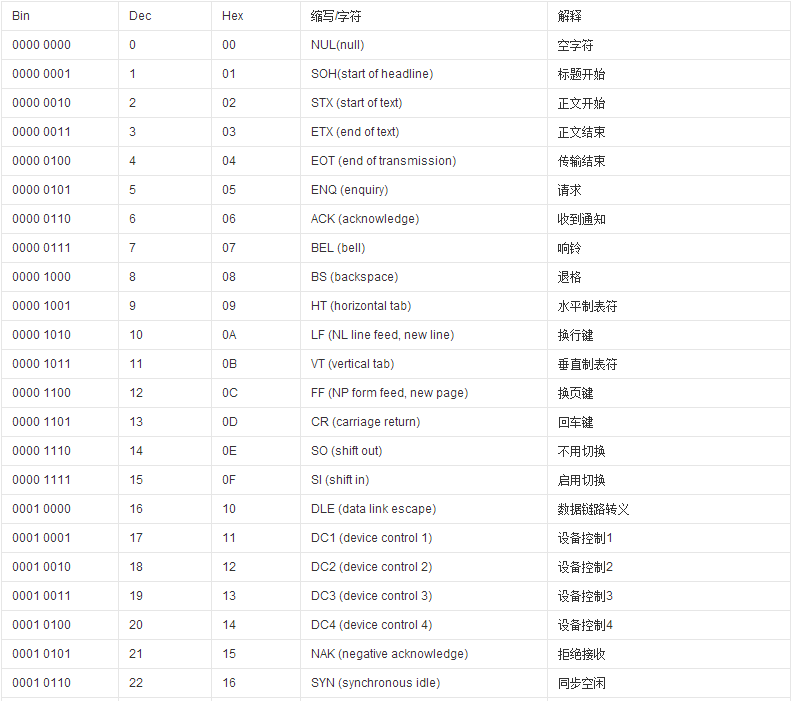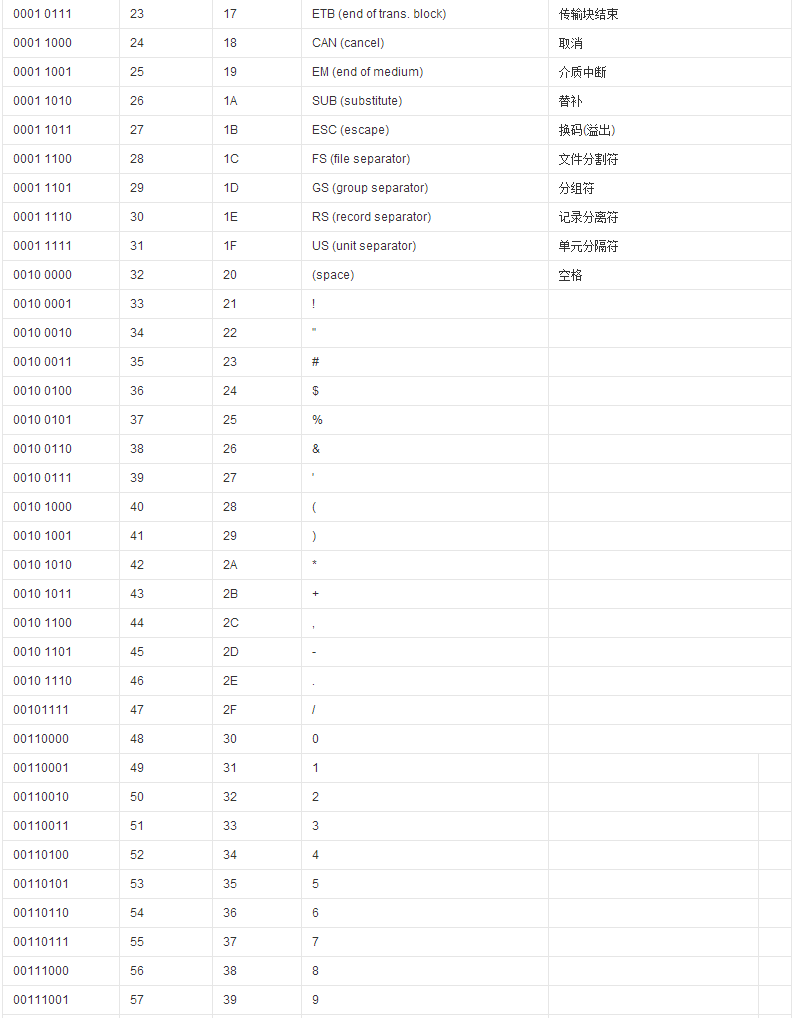我们看到了ASCII是用 8位的二进制 来代表 大小写英文、数字、标点符号、控制符等。 上面我们也说了 8位 二进制 程序里用byte表示 -128 到 127 在ASCII里我们可以看到 最大就是 127 依照上表。我们可以知道 byte可以代表127个ASCII字符。ASCII里包括大小写、数字、符号等。所以我们就知道了。英文、数字、符号等。占用 1 byte 也就是1字节。也就是占用 8个二进制位 我们上面也说了。加密就是让能读懂的、有意义的字符串变成不能读懂、无意义的字符串。好了。我们接下来看。 明文： Hello ASCII： 0100100001100101011011000110110001101111 //上面这就是在ASCII编码下 Hello 在电脑里存放的二进制数据 我们来给拆分一下 让我们看清楚点 01001000 01100101 01101100 01101100 01101111 //也就是 (根据ASCII表对照关系) 01001000 对应 H 01100101 对应 e 01101100 对应 l 01101100 对应 l 01101111 对应 o 我们看了ASCII的对应关系。同时知道不同明文对应的二进制是不一样的。Hello 这是有意义的字符串。我们可以看懂。 我们需要把他变得我们看不懂。我们怎么做？ 这就是加密。不同的操作。不同的加密。对应的就是不同的加密算法。 就如同网上的各种加密算法 BASE64、MD5、DES、RSA 这些。 五：ASCII加密 了解了上面的东西之后。我们知道了 ASCII就是把 8位的二进制数据 转换 成我们能看懂的字符。 然后我们加密。就是要让别人看不懂我原来的数据。 上面也说了Hello的二进制。这里继续说。 要把 “Hello” 这段明文 加密 成让我们不知道这个字符串的原本含义。最简单的是。让他们每个字母都变化。让他加密后的数据不是 “Hello” 这样。我们加密的目的就达到了。 怎么让这串字符串变成不是他自己呢。 这就是具体的算法。 我们这里来随便说一种。 让这段字符串 “Hello” 的每一个字符 都 - 5 我们运用ASCII的表来操作。 H - 5 = C e - 5 =  l - 5 = g l - 5 = g o - 5 = j //加密结果为 Cggj //然后我们来看看 //明文： Hello //密文: Cggj 这样 我们就将Hello 加密成 Cggj了 达到了我们加密的需求（将 有意义 的字符串 转换成 无意义 的字符串 ） 这里我们得加密算法就是 取每一个ASCII字符 将他们都做 - 5 操作 。说了这么多，原因只有一个，那就是让你们理解、明白。。。 好了。你们该说 Talk is cheap. Show me the code 那我就不多废话了。来~ 金刚！ show we code！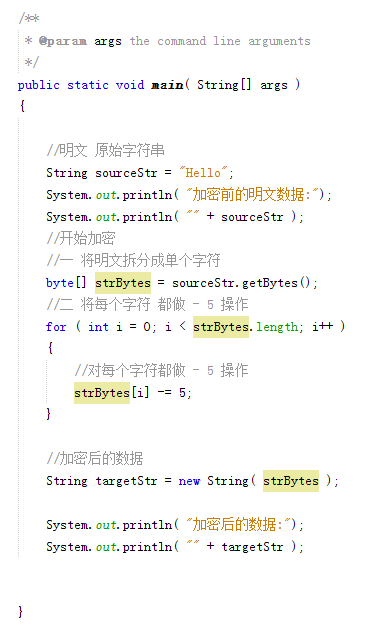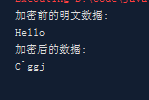发一下文字代码。让大家可以复制下去自己运行 public static void main( String[] args ) { //明文 原始字符串 String sourceStr = "Hello"; System.out.println( "加密前的明文数据:"); System.out.println( "" + sourceStr ); //开始加密 //一 将明文拆分成单个字符 byte[] strBytes = sourceStr.getBytes(); //二 将每个字符 都做 - 5 操作 for ( int i = 0; i < strBytes.length; i++ ) { //对每个字符都做 - 5 操作 strBytes[i] -= 5; } //加密后的数据 String targetStr = new String( strBytes ); System.out.println( "加密后的数据:"); System.out.println( "" + targetStr ); } //运行结果 加密前的明文数据: Hello 加密后的数据: Cggj 这就是最简单的ASCII的一种加密方式。来下面看看常规的加密的用处 //加密前的明文数据: {"name":"brok1n", "age":22, "sex":1, "birthday":"1993-02-28"} //加密后的数据: vi\h5]mjf,i'\b5--'ns5,']dmoc_\t5,44.(+-(-3x //很明显。没加密。我们都能认识看懂、是一串有规则、有意义的字符串。但是加密之后。就成了没有意义的字符串了。 这就是ASCII的简单加密（由于我们刚接触加密我们先只了解这么多。这种加密方式写法还有很多缺点没有完善，但这是加密的最基本的思路。） 下面来看看我们的 解密 六：ASCII解密 说白了。解密就是还原。还原。大家应该都知道了吧。就是把加密的算法。反过来。那么怎么反过来呢。我们接下来看。  //开始解密 //一 将密文拆分成单个字符 byte[] tarBytes = targetStr.getBytes(); //二 将每个字符 都做 + 5 操作 (加密是 -5 我们这里反过来就是 +5) for ( int i = 0; i < tarBytes.length; i++ ) { //对每个字符都做 + 5 (操作 加密是 -5 我们这里反过来就是 +5) tarBytes[i] += 5; } //加密后的数据 String reTarStr = new String( tarBytes ); System.out.println( "解密后的数据:"); System.out.println( "" + reTarStr ); //结果 解密后的数据: {"name":"brok1n", "age":22, "sex":1, "birthday":"1993-02-28"} 以上就是解密。 然后我们来看看整体的代码。以及结果 public static void main( String[] args ) { //明文 原始字符串 //json数据 {"name":"brok1n", "age":22, "sex":1, "birthday":"1993-02-28"} String sourceStr = "{\"name\":\"brok1n\", \"age\":22, \"sex\":1, \"birthday\":\"1993-02-28\"}"; System.out.println( "加密前的明文数据:"); System.out.println( "" + sourceStr ); //开始加密 //一 将明文拆分成单个字符 byte[] strBytes = sourceStr.getBytes(); //二 将每个字符 都做 - 5 操作 for ( int i = 0; i < strBytes.length; i++ ) { //对每个字符都做 - 5 操作 strBytes[i] -= 5; } //加密后的数据 String targetStr = new String( strBytes ); System.out.println( "加密后的数据:"); System.out.println( "" + targetStr ); System.out.println( "解密"); //开始解密 //一 将密文拆分成单个字符 byte[] tarBytes = targetStr.getBytes(); //二 将每个字符 都做 - 5 操作 for ( int i = 0; i < tarBytes.length; i++ ) { //对每个字符都做 - 5 操作 tarBytes[i] += 5; } //加密后的数据 String reTarStr = new String( tarBytes ); System.out.println( "解密后的数据:"); System.out.println( "" + reTarStr ); } 结果如下： 加密前的明文数据: {"name":"brok1n", "age":22, "sex":1, "birthday":"1993-02-28"} 加密后的数据: vi\h5]mjf,i'\b5--'ns5,']dmoc_\t5,44.(+-(-3x 解密 解密后的数据: {"name":"brok1n", "age":22, "sex":1, "birthday":"1993-02-28"}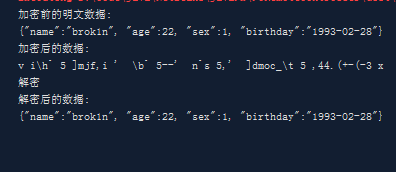好了。这就是ASCII加密最基本的思路。当然这个加密解密已经说了是ASCII加密解密。只能做ASCII表上的那些字符的加密解密。但是这个加密的写法。有很多、很大的问题。比如如果对一个字符做-5操作后他的ASCII小于0 就会出问题。 尾声 好了。我们学习到这里。就应该会明白了我上面说的ASCII中的最简单的加密解密。下一篇。我们继续来学习ASCII的加密解密。来解决这里留下的问题。 展开全文ascii • 案例1： 换位加密解密算法 import java.io.BufferedReader; import java.io.IOException; import java.io.InputStreamReader; import java.util.*; public class demo { static char[] jiami(char[] str, int n) /... 案例1： 换位加密解密算法 import java.io.BufferedReader; import java.io.IOException; import java.io.InputStreamReader; import java.util.*; public class demo { static char[] jiami(char[] str, int n) //加密算法 { int i, j, k, d; int len; char[] temp, miwen, mtemp = {}; len = str.length; //字符串长度 if ((d = len % n) != 0) { len = len + n - d; } if ((temp = new char[len + 1]) == null) //申请内存 { System.out.print("申请内存失败!\n"); System.exit(1); } if ((miwen = new char[len + 1]) == null) //申请内存 { System.out.print("申请内存失败!\n"); System.exit(1); } else { mtemp = miwen; } System.arraycopy(str, 0, temp, 0, str.length); for (i = str.length; i < len; i++) { temp[i] = 32; } temp[len] = '\0'; i = 0; for (k = 0; k < n; k++) //转换，加密 { for (j = 0; j < len / n; j++) { mtemp[i] = temp[k + j * n]; i++; } } mtemp[i] = '\0'; temp = null; return miwen; //返回密文 } static char[] jiemi(char[] str, int n) //解密算法 { int i, j, k = 0, d; int len; char[] temp, mingwen, mtemp = {}; len = str.length; if ((d = len % n) != 0) { len = len - d; } n = len / n; if ((temp = new char[len + 1]) == null) //申请内存 { System.out.print("申请内存失败!\n"); System.exit(1); } if ((mingwen = new char[len + 1]) == null) //申请内存 { System.out.print("申请内存失败!\n"); System.exit(1); } else { mtemp = mingwen; } System.arraycopy(str, 0, temp, 0, str.length); for (i = str.length; i < len; i++) { temp[i] = 32; } temp[len] = '\0'; i = 0; for (k = 0; k < n; k++) //恢复出明文 { for (j = 0; j < len / n; j++) { mtemp[i] = temp[k + j * n]; i++; } } while (mtemp[--i] == 32) ; i++; mtemp[i] = '\0'; return mingwen; //返回明文 } public static void main(String[] args) throws IOException { int n, i; char[] srcstr = new char; char[] miwen; String go; System.out.print("换位加密解密算法演示！\n"); do { System.out.print("\n请先输入换位加密矩阵每行的字符数："); Scanner input = new Scanner(System.in); n = input.nextInt(); //输入换位加密矩阵每行的字符数 System.out.println("请输入明文："); //输入明文字符串 BufferedReader bufferedReader = new BufferedReader(new InputStreamReader(System.in)); String str = bufferedReader.readLine(); srcstr = str.toCharArray(); System.out.print("输入的明文为："); for (i = 0; i < srcstr.length; i++) { System.out.print(srcstr[i]); } miwen = jiami(srcstr, n); //加密 System.out.print("\n加密后的密文为："); for (i = 0; i < srcstr.length; i++) { System.out.print(miwen[i]); } miwen = jiemi(miwen, n); //解密 System.out.print("\n解密出的明文为："); for (i = 0; i < srcstr.length; i++) { System.out.print(miwen[i]); } System.out.print("\n继续执行(y/n)?"); go = input.next(); } while (go.equalsIgnoreCase("y")); System.out.println("演示结束！"); } }  案例2： 替换加密解密算法  import java.io.BufferedReader; import java.io.IOException; import java.io.InputStreamReader; import java.util.Scanner; public class demo { static char[] jiami(char[] str, int n) //加密算法 { int i, len; char[] miwen; len = str.length; if ((miwen = new char[len + 1]) == null) //申请内存 { System.out.print("申请内存失败!\n"); System.exit(1); } for (i = 0; i < len; i++) //移位替换 { miwen[i] = (char) (str[i] + n); } miwen[len] = '\0'; return miwen; } static char[] jiemi(char[] str, int n) //解密算法 { int i, len; char[] mingwen; len = str.length; if ((mingwen = new char[len + 1]) == null)//申请内存 { System.out.print("申请内存失败!\n"); System.exit(1); } for (i = 0; i < len; i++) //移位替换 { mingwen[i] = (char) (str[i] - n); } mingwen[len] = '\0'; return mingwen; } public static void main(String[] args) throws IOException { int i, n; char[] srcstr = new char; char[] miwen; String go; System.out.print("替换加密解密算法演示！\n"); do { System.out.print("\n请先输入替换加密解密算法中的密钥："); Scanner input = new Scanner(System.in); n = input.nextInt(); //密钥 System.out.print("请输入明文字符串："); BufferedReader bufferedReader = new BufferedReader(new InputStreamReader(System.in)); String str = bufferedReader.readLine(); srcstr = str.toCharArray(); //明文字符串 System.out.print("输入的明文为："); for (i = 0; i < srcstr.length; i++) { System.out.print(srcstr[i]); } miwen = jiami(srcstr, n); //加密 System.out.print("\n加密后的密文为："); for (i = 0; i < srcstr.length; i++) { System.out.print(miwen[i]); } miwen = jiemi(miwen, n); //解密 System.out.print("\n解密出的明文为："); for (i = 0; i < srcstr.length; i++) { System.out.print(miwen[i]); } System.out.print("\n继续执行(y/n)?"); go = input.next(); } while (go.equalsIgnoreCase("y")); System.out.println("演示结束！"); } }  执行结果如下：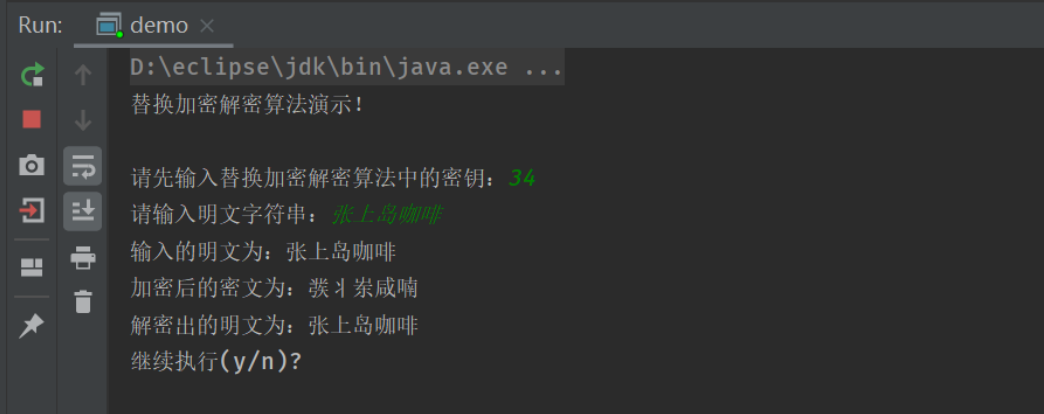案例3：位加密解密算法 import java.io.BufferedReader; import java.io.IOException; import java.io.InputStreamReader; import java.util.Scanner; public class demo { static char[] bitcode(char[] str, char n) //位加密解密算法 { int i, len; char[] wen; len = str.length; if ((wen = new char[len + 1]) == null) { System.out.print("申请内存失败!\n"); System.exit(1); } for (i = 0; i < len; i++) { wen[i] = (char) (str[i] ^ n); //异或运算 } wen[len] = '\0'; return wen; } public static void main(String[] args) throws IOException { int i; char ch; char[] srcstr = new char; char[] miwen; String go; System.out.print("位加密解密算法演示！\n"); do { System.out.print("\n请先输入替换加密解密算法中的密钥："); Scanner input = new Scanner(System.in); ch = input.next().charAt(0); //密钥 System.out.print("请输入明文字符串："); BufferedReader bufferedReader = new BufferedReader(new InputStreamReader(System.in)); String str = bufferedReader.readLine(); srcstr = str.toCharArray(); //明文字符串 System.out.print("输入的明文为："); for (i = 0; i < srcstr.length; i++) { System.out.print(srcstr[i]); } miwen = bitcode(srcstr, ch); //加密 System.out.print("\n加密后的密文为："); for (i = 0; i < srcstr.length; i++) { System.out.print(miwen[i]); } miwen = bitcode(miwen, ch); //解密 System.out.print("\n解密出的明文为："); for (i = 0; i < srcstr.length; i++) { System.out.print(miwen[i]); } System.out.print("\n继续执行(y/n)?"); go = input.next(); } while (go.equalsIgnoreCase("y")); System.out.println("演示结束！"); } }  执行结果如下：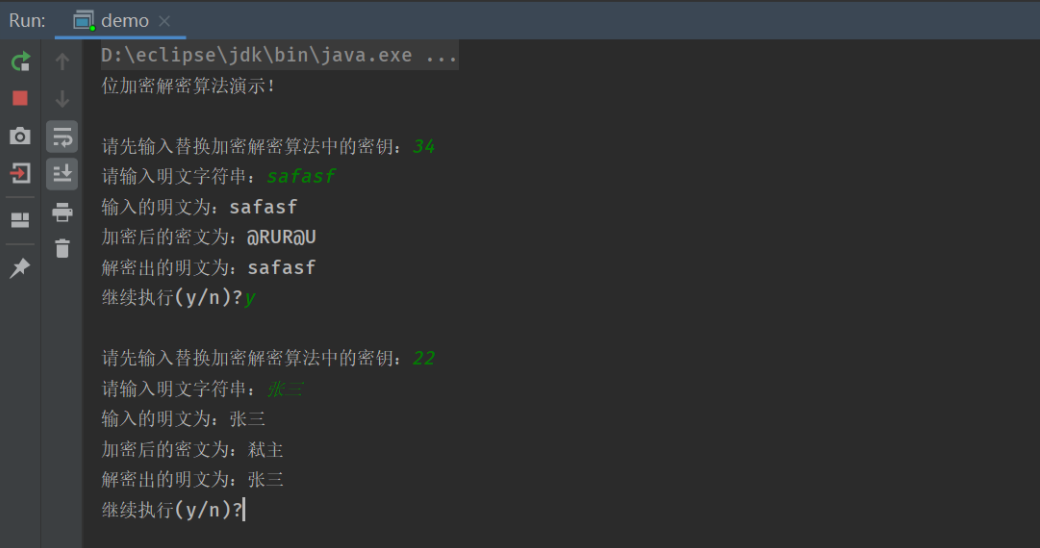案例4： 一次一密加密算法 import java.io.BufferedReader; import java.io.IOException; import java.io.InputStreamReader; import java.util.Random; import java.util.Scanner; public class demo { static int MAX = 100; static char[] key = new char[MAX]; //用于保存密钥 static int len; static char[] bitcode(char[] str) //一次一密加密算法 { int i; char[] wen; if ((wen = new char[len + 1]) == null) { System.out.printf("申请内存失败!\n"); System.exit(1); } for (i = 0; i < len; i++) { wen[i] = (char) (str[i] ^ key[i]); //异或运算 } wen[len] = '\0'; return wen; } public static void main(String[] args) throws IOException { int i; char[] srcstr = new char[MAX]; char[] miwen, mingwen; String go; Scanner input = new Scanner(System.in); // srand(time(NULL)); //随机种子 System.out.printf("一次一密加密解密算法演示！\n"); do { System.out.printf("请输入明文字符串："); BufferedReader bufferedReader = new BufferedReader(new InputStreamReader(System.in)); String str = bufferedReader.readLine(); srcstr = str.toCharArray(); //明文信息 Random r = new Random(); //随机种子 len = srcstr.length; for (i = 0; i < len; i++) { key[i] = (char) (r.nextInt(10) + '0'); //产生密钥序列 } System.out.printf("此次加密解密演示的密钥序列为："); for (i = 0; i < len; i++) { System.out.printf("%c", key[i]); } // System.out.printf("\n"); miwen = bitcode(srcstr); //加密 System.out.printf("\n输入的明文为："); for (i = 0; i < srcstr.length; i++) System.out.print(srcstr[i]); System.out.printf("\n加密后的密文为："); for (i = 0; i < miwen.length; i++) System.out.print(miwen[i]); mingwen = bitcode(miwen); //解密 System.out.printf("\n解密出的明文为："); for (i = 0; i < mingwen.length; i++) System.out.print(mingwen[i]); System.out.print("\n\n继续执行(y/n)?"); go = input.next(); } while (go.equalsIgnoreCase("y")); System.out.println("演示结束！"); } }  执行结果如下：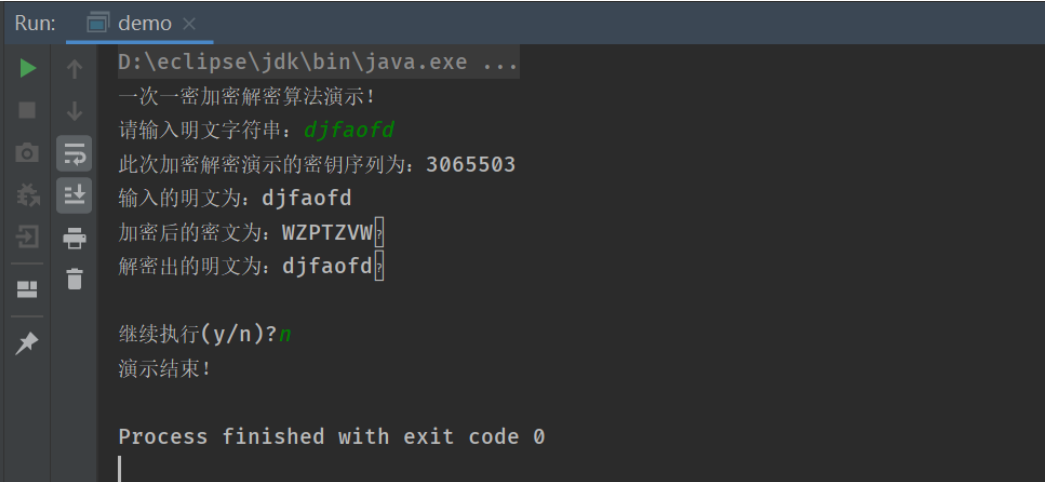` 展开全文• ## Java实现DES加密解密 万次阅读 多人点赞 2018-11-09 16:27:01 DES(Data Encryption Standard)是一种对称加密算法，所谓对称加密就是加密解密都是使用同一个密钥。 加密原理： DES 使用一个 56 位的密钥以及附加的 8 位奇偶校验位，产生最大 64 位的分组大小。这是一个迭代的...DES • 普通hash函数如md5、sha1、base64等都是不可逆函数。...但是跨语言时这类可逆函数非常难搞定。所以这时尽量使用AES ...包含超时的加密解密函数 /** * 加密 * @param string$string 要加密或解密
• 课程内容包含乱码的原因分析、代码的压缩与优化、JavaScript/html前端代码加密解密、前端加密防360浏览器拦截、html代码解密原理分析、万能解密神器、手动解密html代码等等。
• ## sm4算法加密解密

万次阅读 2020-05-08 09:58:52
它将明文分成多个等长的模块（block），使用确定的算法和对称密钥对每组分别加密解密。 对称加密、加密算法主要可以分为两种，一个是非对称加密算法，另一个就是对称加密算法。对称加密简单来说就是有一个明文，...sm4
• DES加密共有四种模式：电子密码本模式（ECB）、加密分组链接模式（CBC）、加密反馈模式（CFB）和输出反馈模式（OFB）。 DES加密的CBC模式加密解密和ECB模式加密解密android平台
• 培根在线解码 https://tool.bugku.com/peigen/ AABBAABBBAABBBAAAABBABBABABAAAAABBAAABBBBAABBBAABABABBAAABAAAABAABAABBABAAAAABAA ... 指纹识别： ... md5在线解密： https://pmd5.com/?mType=Group ...python
• 一款简单不错的png加密解密工具，手机游戏有的PNG图被加密，可用这工具解密
• 之前上传的有ECC密钥生成、加密解密、签名验签工具，忘了放源码了，这个是源代码，VS2010的，由于上传大小有限制，所以分开了，这个是加密解密
• ## Android RSA加密解密demo

千次下载 热门讨论 2014-08-23 14:37:48
Android RSA加密解密demo，详情请参看博客：http://blog.csdn.net/bbld_/article/details/38777491
• 这类加密，可大量加大源码文件，不但导致无法正常阅读，更大影响服务器性能。而且阻碍二次修改开发。...这类加密解密难度相对较大，但也可以解密 如需付费解密　可以联系q 945912362 添加前请说明csdn　sg解密 ...
• ## SpringBoot中接口加密解密统一处理

万次阅读 多人点赞 2019-07-27 19:49:38
我们与客户端的接口交互中，为了更高的安全性，我们可能需要对接口加密（请求参数加密，服务端解密）、返回信息加密（服务端加密，客户端...将接口参数的加密解密和返回信息的加密解密分开，分别定义注解，利用C...SpringBoot 注解
• 谈谈对通信加密解密的理解。 加密解密分为：对称可逆加密，非对称可逆加密两大类； 可逆加密：加密后得到密文，可以通过加密后的密文得到原文； 对称可逆加密特点：有一个公开的加密算法，任何人都知道；有一组Key，...
• ## C#DES加密解密

万次阅读 2017-04-13 19:57:59
关于DES加密解密由于项目中用到了,主要用在了对登录的用户名进行加密解密。一个程序某些关键信息肯定是要有安全性要求的，这个时候其实就需要加密和解密的过程，保障起安全性。 DES，全称Data Encryption ...
• C#做的RSA加密解密， 带winform界面， 测试通过， 代码完整。
• ## 异或加密解密

万次阅读 2019-05-26 19:19:51
异或加密解密 异或规则 同为0，异为1； 一个数和另外一个数进行两次异或后，是原数本身。如下例 a -01100001 3 -00000011 01100010 3 -00000011 01100001 异或加密解密规则 数据进行异或一次，加密。...
• ## 配置文件加密解密

万次阅读 2020-10-10 17:04:26
配置文件中存放着许多重要的东西，比如数据库账号密码什么的，需要对这些内容进行加密储存。 使用到的是jasypt 1.添加Maven依赖 <dependency> <groupId>com.github.ulisesbocchio</groupId> <...
• ## 希尔密码加密解密

万次阅读 2018-09-09 17:04:30
希尔密码加密解密原理: 希尔密码是运用基本矩阵论原理的替换密码。每个字母当作26进制数字：A=0，B=1...一串字母当成n维向量，跟一个n×n的矩阵相乘，再将得出的结果MOD 26。注意用作加密的矩阵（即密钥）必须是...
• ## 栅栏密码加密解密

热门讨论 2012-05-27 12:07:44
栅栏密码加密解密，26栏以上通过测试，不必整除栏数，可以任意加密解密。 MV 开源设计工作室
• Delphi 各种字符串的加密解密方法，含括2007版本和2012版本的加密解密方法
• 楼上兄弟太黑，特意找的源码，2分处理，完美解决Java加密解密zip文件
• ## AES128 加密解密 C#

热门讨论 2013-01-04 15:36:24
AES128 加密 解密 C#实现 可以实现文件等的加密解密...